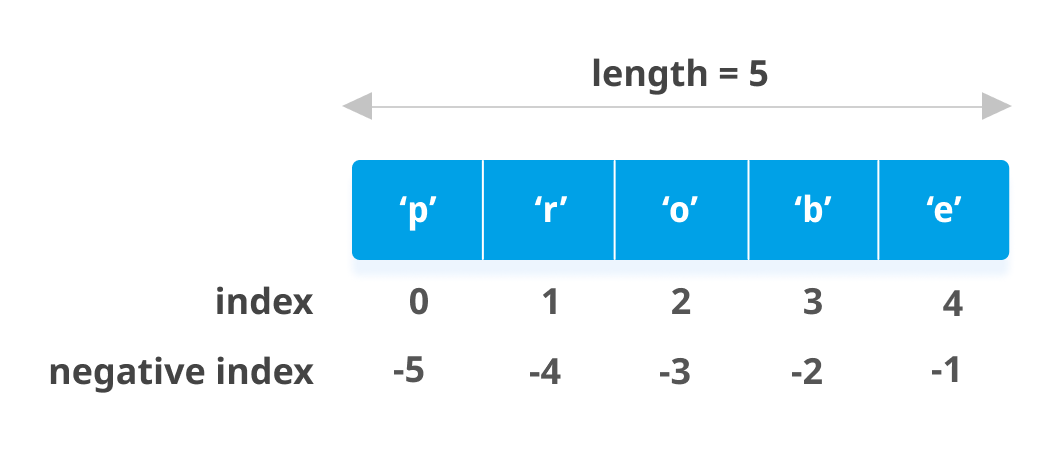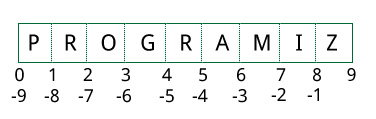# Python List

In this article, we'll learn everything about Python lists; how they are created, slicing of a list, adding or removing elements from them and so on.

Python offers a range of compound datatypes often referred to as sequences. List is one of the most frequently used and very versatile datatype used in Python.

## How to create a list?

In Python programming, a list is created by placing all the items (elements) inside a square bracket [ ], separated by commas.

It can have any number of items and they may be of different types (integer, float, string etc.).

``````# empty list
my_list = []

# list of integers
my_list = [1, 2, 3]

# list with mixed datatypes
my_list = [1, "Hello", 3.4]
``````

Also, a list can even have another list as an item. This is called nested list.

```# nested list
my_list = ["mouse", [8, 4, 6], ['a']]
```

## How to access elements from a list?

There are various ways in which we can access the elements of a list.

### List Index

We can use the index operator [] to access an item in a list. Index starts from 0. So, a list having 5 elements will have index from 0 to 4.

Trying to access an element other that this will raise an `IndexError`. The index must be an integer. We can't use float or other types, this will result into `TypeError`.

Nested list are accessed using nested indexing.

``````my_list = ['p','r','o','b','e']
# Output: p
print(my_list)

# Output: o
print(my_list)

# Output: e
print(my_list)

# Error! Only integer can be used for indexing
# my_list[4.0]

# Nested List
n_list = ["Happy", [2,0,1,5]]

# Nested indexing

# Output: a
print(n_list)

# Output: 5
print(n_list)
``````

### Negative indexing

Python allows negative indexing for its sequences. The index of -1 refers to the last item, -2 to the second last item and so on.

``````my_list = ['p','r','o','b','e']

# Output: e
print(my_list[-1])

# Output: p
print(my_list[-5])
``````## How to slice lists in Python?

We can access a range of items in a list by using the slicing operator (colon).

``````my_list = ['p','r','o','g','r','a','m','i','z']
# elements 3rd to 5th
print(my_list[2:5])

# elements beginning to 4th
print(my_list[:-5])

# elements 6th to end
print(my_list[5:])

# elements beginning to end
print(my_list[:])
``````

Slicing can be best visualized by considering the index to be between the elements as shown below. So if we want to access a range, we need two indices that will slice that portion from the list.## How to change or add elements to a list?

List are mutable, meaning, their elements can be changed unlike string or tuple.

We can use assignment operator (=) to change an item or a range of items.

``````# mistake values
odd = [2, 4, 6, 8]

# change the 1st item
odd = 1

# Output: [1, 4, 6, 8]
print(odd)

# change 2nd to 4th items
odd[1:4] = [3, 5, 7]

# Output: [1, 3, 5, 7]
print(odd)
``````

We can add one item to a list using `append()` method or add several items using `extend()` method.

``````odd = [1, 3, 5]

odd.append(7)

# Output: [1, 3, 5, 7]
print(odd)

odd.extend([9, 11, 13])

# Output: [1, 3, 5, 7, 9, 11, 13]
print(odd)
``````

We can also use + operator to combine two lists. This is also called concatenation.

The * operator repeats a list for the given number of times.

``````odd = [1, 3, 5]

# Output: [1, 3, 5, 9, 7, 5]
print(odd + [9, 7, 5])

#Output: ["re", "re", "re"]
print(["re"] * 3)
``````

Furthermore, we can insert one item at a desired location by using the method `insert()` or insert multiple items by squeezing it into an empty slice of a list.

``````odd = [1, 9]
odd.insert(1,3)

# Output: [1, 3, 9]
print(odd)

odd[2:2] = [5, 7]

# Output: [1, 3, 5, 7, 9]
print(odd)
``````

## How to delete or remove elements from a list?

We can delete one or more items from a list using the keyword `del`. It can even delete the list entirely.

``````my_list = ['p','r','o','b','l','e','m']

# delete one item
del my_list

# Output: ['p', 'r', 'b', 'l', 'e', 'm']
print(my_list)

# delete multiple items
del my_list[1:5]

# Output: ['p', 'm']
print(my_list)

# delete entire list
del my_list

# Error: List not defined
print(my_list)
``````

We can use `remove()` method to remove the given item or `pop()` method to remove an item at the given index.

The `pop()` method removes and returns the last item if index is not provided. This helps us implement lists as stacks (first in, last out data structure).

We can also use the `clear()` method to empty a list.

``````my_list = ['p','r','o','b','l','e','m']
my_list.remove('p')

# Output: ['r', 'o', 'b', 'l', 'e', 'm']
print(my_list)

# Output: 'o'
print(my_list.pop(1))

# Output: ['r', 'b', 'l', 'e', 'm']
print(my_list)

# Output: 'm'
print(my_list.pop())

# Output: ['r', 'b', 'l', 'e']
print(my_list)

my_list.clear()

# Output: []
print(my_list)
``````

Finally, we can also delete items in a list by assigning an empty list to a slice of elements.

``````>>> my_list = ['p','r','o','b','l','e','m']
>>> my_list[2:3] = []
>>> my_list
['p', 'r', 'b', 'l', 'e', 'm']
>>> my_list[2:5] = []
>>> my_list
['p', 'r', 'm']
``````

## Python List Methods

Methods that are available with list object in Python programming are tabulated below.

They are accessed as `list.method()`. Some of the methods have already been used above.

Some examples of Python list methods:

``````my_list = [3, 8, 1, 6, 0, 8, 4]

# Output: 1
print(my_list.index(8))

# Output: 2
print(my_list.count(8))

my_list.sort()

# Output: [0, 1, 3, 4, 6, 8, 8]
print(my_list)

my_list.reverse()

# Output: [8, 8, 6, 4, 3, 1, 0]
print(my_list)
``````

## List Comprehension: Elegant way to create new List

List comprehension is an elegant and concise way to create new list from an existing list in Python.

List comprehension consists of an expression followed by for statement inside square brackets.

Here is an example to make a list with each item being increasing power of 2.

``````pow2 = [2 ** x for x in range(10)]

# Output: [1, 2, 4, 8, 16, 32, 64, 128, 256, 512]
print(pow2)
``````

This code is equivalent to

``````pow2 = []
for x in range(10):
pow2.append(2 ** x)
``````

A list comprehension can optionally contain more `for` or if statements. An optional `if` statement can filter out items for the new list. Here are some examples.

``````>>> pow2 = [2 ** x for x in range(10) if x > 5]
>>> pow2
[64, 128, 256, 512]
>>> odd = [x for x in range(20) if x % 2 == 1]
>>> odd
[1, 3, 5, 7, 9, 11, 13, 15, 17, 19]
>>> [x+y for x in ['Python ','C '] for y in ['Language','Programming']]
['Python Language', 'Python Programming', 'C Language', 'C Programming']
``````

## Other List Operations in Python

### List Membership Test

We can test if an item exists in a list or not, using the keyword `in`.

``````my_list = ['p','r','o','b','l','e','m']

# Output: True
print('p' in my_list)

# Output: False
print('a' in my_list)

# Output: True
print('c' not in my_list)
``````

### Iterating Through a List

Using a `for` loop we can iterate though each item in a list.

``````for fruit in ['apple','banana','mango']:
print("I like",fruit)```
```